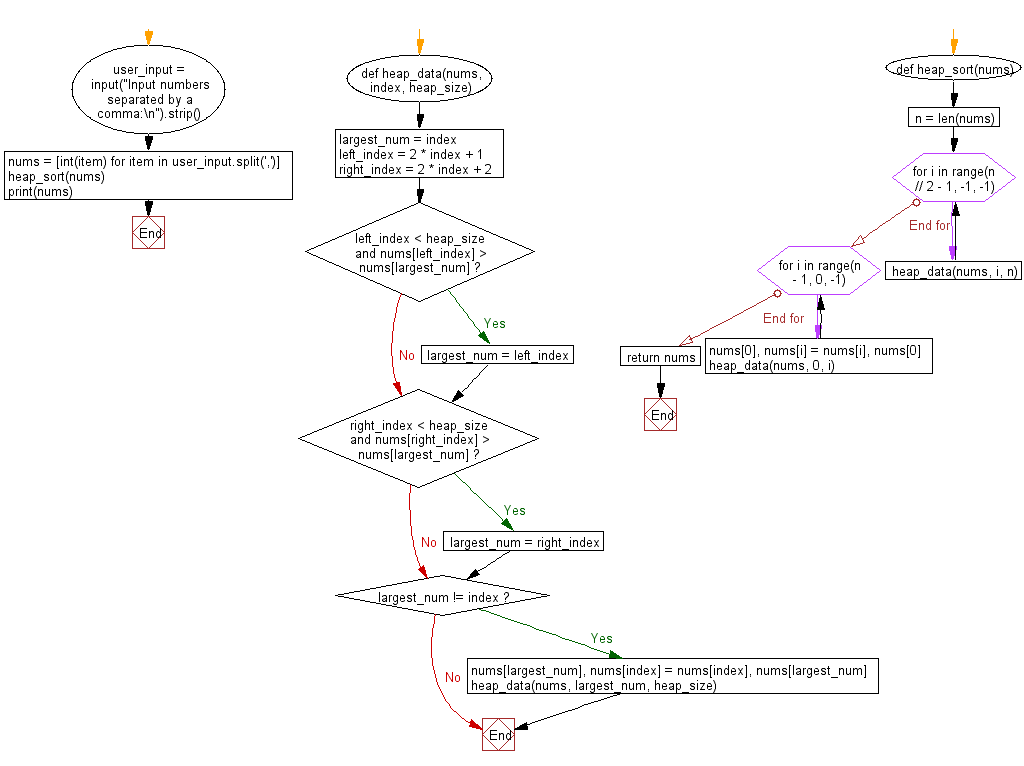﻿ Python: Sort a list of elements using Heap sort - w3resource# Python: Sort a list of elements using Heap sort

## Python Search and Sorting : Exercise-17 with Solution

Write a Python program to sort a list of elements using Heap sort.
In computer science, heapsort (invented by J. W. J. Williams in 1964) is a comparison-based sorting algorithm. Heapsort can be thought of as an improved selection sort: like that algorithm, it divides its input into a sorted and an unsorted region, and it interactively shrinks the unsorted region by extracting the largest element and moving that to the sorted region. The improvement consists of the use of a heap data structure rather than a linear-time search to find the maximum. Although somewhat slower in practice on most machines than a well-implemented quicksort, it has the advantage of a more favorable worst-case O(n log n) runtime. Heapsort is an in-place algorithm, but it is not a stable sort. A run of the heapsort algorithm sorting an array of randomly permuted values. In the first stage of the algorithm the array elements are reordered to satisfy the heap property. Before the actual sorting takes place, the heap tree structure is shown briefly for illustration.

Sample Solution:

Python Code:

``````def heap_data(nums, index, heap_size):
largest_num = index
left_index = 2 * index + 1
right_index = 2 * index + 2
if left_index < heap_size and nums[left_index] > nums[largest_num]:
largest_num = left_index

if right_index < heap_size and nums[right_index] > nums[largest_num]:
largest_num = right_index
if largest_num != index:
nums[largest_num], nums[index] = nums[index], nums[largest_num]
heap_data(nums, largest_num, heap_size)
def heap_sort(nums):
n = len(nums)
for i in range(n // 2 - 1, -1, -1):
heap_data(nums, i, n)
for i in range(n - 1, 0, -1):
nums, nums[i] = nums[i], nums
heap_data(nums, 0, i)
return nums
user_input = input("Input numbers separated by a comma:\n").strip()
nums = [int(item) for item in user_input.split(',')]
heap_sort(nums)
print(nums)
```
```

Sample Output:

```Input numbers separated by a comma:
15, 79, 28, 35, 68
[15, 28, 35, 68, 79]
```

Flowchart:Python Code Editor:

What is the difficulty level of this exercise?

Test your Programming skills with w3resource's quiz.

﻿

## Python: Tips of the Day

Use Reversed() In for Loops:

```>>> tasks = ['laundry', 'picking up kids', 'gardening', 'cooking']